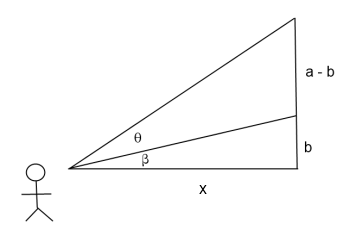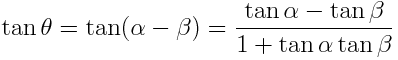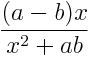# Solution to Renaissance problem

The previous post presented a problem first posed by Johannes Müller in 1471.

Where you should stand so that a vertical bar appears longest?

To be more precise, suppose a vertical bar is hanging so that the top of the bar is a distance a above your eye level and the bottom is a distance b above your eye level. Let x be the horizontal distance to the bar. For what value of x does the bar appear longest?

In the following diagram, we wish to maximize the angle θ.Since tangent is an increasing function, it suffices to maximize tan(θ). Let α = θ + β. ThenNow use tan α = a/x and tan β = b/x to reduce the expression above toNow we have a function of x to maximize. Take the derivative and find where it is zero. The maximum occurs at √ab, the geometric mean of a and b.

However, when Müller proposed his problem in 1471, calculus had not yet been invented, so we can be pretty sure Müller did not take derivatives. I don’t know how (or even if) Müller solved his problem, but the book where I found the problem showed how it could be solved without calculus. The derivation is a little longer, but it only depends on simple algebra and the arithmetic-geometric mean inequality, i.e. the observation that (a + b) /2 ≥ √ab.

Update: Here is a purely geometric solution by George Papademetriou.

Update: See this post for more historical background.

Other posts about the geometric mean:

## 11 thoughts on “Solution to Renaissance problem”

1. Nick Craig-Wood

That is the answer I got too in pretty much the same way (trig identities and a bit of calculus) – nice problem! (Thanks also to Wolfram Alpha which knows a lot more calculus than I can remember ;-)

The fact that the answer is so simple made me think that there was probably a non-calculus solution but I couldn’t think of it…

2. Jan Van lent

Maximizing the angle directly is also fairly straightforward since the derivative of the inverse tangent is a rational function. You do not need the identity for the tangent of a sum in this case. The derivative of the angle becomes b/(x^2+b^2)-a/(x^2+a^2).

3. Mueller did not know Calculus, but I bet he did know about conics, and there is a single circle that passes through the top and bottom of the picture that is also tangent to the horizontal line x, and I think that point will be the point of
“best viewing”.

Our two points for the bar endpoints are A and B, think of them as vertically aligned as in the above picture. Pick a value for angle theta, and consider the locus of points P to the left of the line AB such that the angle APB equals theta. From elementary geometry, this is is an arc of a circle with endpoints A and B. If theta is a right angle, then this is the semicircle connecting A and B. As theta gets smaller, the circle gets larger and its center moves to the left while remaining on the perpendicular bisector of A and B. We have constructed a set of circle arcs of varying radius, all intersecting A and B and covering the half-plane to the left of AB.

Now consider the horizontal half-line that starts on the line AB at a point outside the segment AB and extends to the left perpendicularly to AB. We can identify the angle theta for any point on this line by considering which of the previously constructed family of circles intersects the point. Which point on this half-line has the largest theta? It is the point which intersects the smallest circle in the family. It is evident that this is the unique circle that is tangent to the horizontal half-line and intersects A and B, and that this defines the point uniquely.

Then using the notation of the diagram in the post above, the circle has radius (a+b)/2. If the center of the circle is O, then angle AOB is 2 theta. Divide triangle AOB into two right triangles, hypotenuse (a+b)/2, side (a-b)/2, Pythagoras gives the answer sqrt(ab) for x.

5. Jan Van lent

For some other geometrical constructions of the geometric mean so for example Ch. 2 of The Story of sqrt(-1) by Paul Nahin.

6. lvps1000vm

This problem is solvable with compass and straightedge. Pat Ballew got the right answer, but not the explanation.

Given a circumference and one of its chords, all points of the circumference “see” the chord with the same angle (called the inscribed angle). This angle is the double of the central angle (the angle “seen” by the center of the circumference). Therefore the maximal incribed angle corresponds to the circumference that passes trough the endpoints with the center as close as possible.

Draw the circumference that passes through the endpoints and is tangent to the floor, and the tangency point is the best ‘observation’ point.